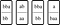# Full Proof that C++ Grammar is Undecidable

Most programming languages’ grammars fall into the category of Context-Free Grammar (CFG), or sometimes Context-Sensitive Grammar (CSG). What about C++? It turns out parsing C++’s grammar is literally undecidable. In other words, if you have a compiler that parses all valid C++ sources perfectly, you have solved the Halting Problem.

To prove this, we will write a program that is parsed differently depending on the solution to the Halting Problem. But we need to implement a Turing Machine to formulate the Halting Problem, and doing that with only template metaprogramming is a huge pain, so we will instead use the Post Correspondence Problem which is proven to be equivalent to the Halting Problem.

The Post Correspondence Problem’s statement is simple: You are given a set of dominoes which have an array of symbols written on the top part and the bottom part. Can you arrange the dominoes, allowing duplicates, so that the string of symbols on the top part matches the string of symbols on the bottom part?Three types of dominoes [bba, bb], [ab, aa], [a, baa] arranged so that the top part “bbaabbbaa” reads the same as the bottom part.

Turns out, there exists no algorithm that says “yes” or “no” to the Post Correspondence Problem in finite time, given any set of dominoes as input.

We first define a variadic struct template `Row` , which represents a “row” of symbols (`int` s). We add a convenience static member constant that says whether this row is empty.

Now we define a template that represents a domino:

And a handy type alias that concatenates two `Row` s together:

Now, to exhaustively search through the entire search space, we will use a Breadth-First Search (BFS) algorithm. In the core of BFS lies a FIFO queue, so we first implement that:

Each state of the search space consists of the upper row and lower row of the already-arranged dominoes. We can check if the state is a match by checking if the two rows are identical and not empty.

Two rows are a “partial match” if the shorter one is the prefix of the longer. This is used to prune the branches where expanding more would be useless:

Now we implement the core of the algorithm. First, pop the head of the queue, and check if the popped state is a match. If it is, the problem is solved, and the answer is “yes”; if it isn’t, but going further could lead us to a solution, push all the child states generated by appending a domino at the right end, and keep going. If the queue is somehow empty, that means we looked at the entire search space and did not find a match, so the answer is “no”.

The initial state is two empty rows:

Now, `DominoList::match` has the solution to the Post Correspondence Problem defined by the template argument `Dominos` !

We can now define a helper struct `ParseThis` whose member `typeOrValue` is a type `int` if the solution to the Post Correspondence Problem is “yes”, and a value `0` of type `int` if the solution is “no”, using SFINAE:

Therefore, the following line is a function declaration if the solution to the Post Correspondence Problem with the dominoes [bba, bb], [ab, aa], [a, baa] is “Yes”, and a variable declaration if it is “No”. (In the former case, it is equivalent to `int x(int);` , and in the latter case, it is equivalent to `int x(0);` or `int x = 0;` )

There we have it! We can substitute any set of dominoes in the above line, and a conforming compiler should be able to decide whether `x` is a function or variable! If such compiler exists, however, we can use it to solve the Post Correspondence Problem for any input, which means we can use it to solve the Halting Problem. But no such program can exist, which means parsing C++ is undecidable. QED